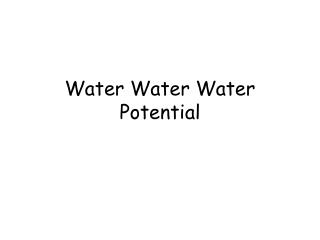DownloadDownload PresentationWater Water Water Potential

# Water Water Water Potential

Télécharger la présentation## Water Water Water Potential

- - - - - - - - - - - - - - - - - - - - - - - - - - - E N D - - - - - - - - - - - - - - - - - - - - - - - - - - -
##### Presentation Transcript

1. Water Water Water Potential

2. What are you water potential? • Measures the tendency of water to leave one place in favor of another • Measured in bars • High water potential = water molecules can easily move about • Low water potential = water molecules cannot easily move about

3. Water Potential • Pure water  water potential of zero • Determines where water is going to flow due to • Osmosis • Gravity • Pressure • Surface tension

4. So What Does This Mean? • The solvent is pulling the water toward it • High solute concentration = low water potential • Low solute concentration = high water potential

5. Review • Moves from hypotonic to hypertonic

6. Review • Hypotonic • Solution= less solute; more water • Cell= more solute; less water • Hypertonic • Solution= more solute; less water • Cell= less solute; more water

7. Temperature • High temperature = more water potential • Why? • Low temperature = less water potential • Why? Water molecules can move easily Water molecules cannot move easily

8. What Will Happen? • When you put a pipette in water? • What do you have to do to get the water out? Water will go naturally up, against gravity, without pressure (-) Put pressure on it (+)

9. Water • Concentration of solute is related to osmotic pressure… • Pressure related to pressure potential… • Pressure raises water potential

10. The 3 Factors • What affects water potential? • Temperature • Solutes • Pressure

11. ψp Pressure Potential • A force that resists water flow due to a container (cell) being full. • Important in plant cells because they are surrounded by a cell wall • Water enters plant cell, its volume increases and the living part of the cell presses on the cell wall

12. ψp Pressure Potential • The cell wall gives very little so pressure builds up…oh no! • This has the tendency to stop more water entering the cell and stops cell from bursting • When plant cell is inflated with water, it is turgid • Pressure potential is called turgor pressure

13. Solute (osmotic) potential (ψs)= –iCRT Yikes, what's that?????? Example Problem: The molar concentration of a sugar solution in an open beaker has been determined to be 0.3M. Calculate the solute potential at 27°C degrees. Round your answer to the nearest hundredth. What is the water potential?

14. Molarity

15. Be very careful to distinguish between moles and molarity. "Moles" measures the amount or quantity of material you have; "molarity" measures the concentration of that material. So when you're given a problem or some information that says the concentration of the solution is 0.1 M that means that it has 0.1 mole for every liter of solution; it does not mean that it is 0.1 moles

16. What is the molarity of a solution made by dissolving 2.5 g of NaCl in enough water to make 125 ml of solution? 1 mole of any substance has a mass = to the atom’s atomic mass. Molarity is number of moles per Liters of solution

17. EXAMPLE PROBLEMS 1- An AP Biology student dissolves 98.4 g of FeSO4 in enough water to make 2.000 L of solution. What is the molarity of the solution? 0.324 M 1 mole . 151.91g x 98.4g = 0.647 moles ÷ 2.00L= 0.324 mol/L 151.91g

18. EXAMPLE PROBLEMS • Calculate the solute potential of a .1M NaCl solution at 22 degrees Celsius. • If the concentration of NaCl inside the plant cell is .15M, which way will the water flow if the cell is placed in the .1M solution?